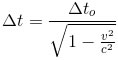﻿ All the formulas related to Theory Of Relativity

# List of formulas related to Theory Of Relativity

#### Mass-Energy Equivalence

m: mass (kg), E: energy of object (J), c: speed of light (m/s)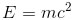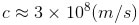#### Length Contraction

lo: real length of object, l: length observed by an observer moving with speed v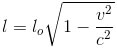#### Relativistic Mass

mo: rest mass, m: relativistic mass of object when moving with speed v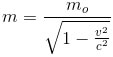#### Time Dilation